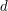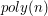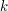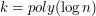## Пятница 8 июля, 18-00, ауд. 106 (совместное заседание с лабораторией им. Чебышева СПбГУ)

Пятница, 8 июля, ауд. 106. Начало в 18:00.

Докладчик: Mark Braverman (University of Toronto).

Тема: Poly-logarithmic independence fools bounded depth circuits.

### Abstract

A Boolean circuit of depthis a circuit comprised of AND, OR and NOT gates arranged in at mostlayers. This class of circuits is one of the few complexity classes where unconditional lower bounds, i.e. computational impossibility results exist. Many of the bounds follow from a deep connection between bounded-depth circuits and low-degree multivariate polynomials.

In this talk we will discuss some of these connections. We will then present a proof of the 1990 Linial-Nisan conjecture on the computational power of bounded-depth circuits. The conjecture stated that bounded-depth Boolean circuits of sizecannot distinguish inputs drawn from a-wise independent distributions from uniform inputs, where. The talk will be almost completely self-contained.